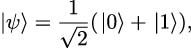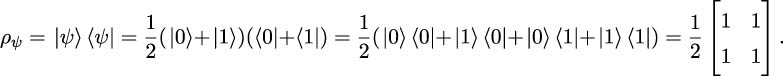•#### Quantum Machine Learning and Optimisation in Finance#### Overview of this book

With recent advances in quantum computing technology, we finally reached the era of Noisy Intermediate-Scale Quantum (NISQ) computing. NISQ-era quantum computers are powerful enough to test quantum computing algorithms and solve hard real-world problems faster than classical hardware. Speedup is so important in financial applications, ranging from analysing huge amounts of customer data to high frequency trading. This is where quantum computing can give you the edge. Quantum Machine Learning and Optimisation in Finance shows you how to create hybrid quantum-classical machine learning and optimisation models that can harness the power of NISQ hardware. This book will take you through the real-world productive applications of quantum computing. The book explores the main quantum computing algorithms implementable on existing NISQ devices and highlights a range of financial applications that can benefit from this new quantum computing paradigm. This book will help you be one of the first in the finance industry to use quantum machine learning models to solve classically hard real-world problems. We may have moved past the point of quantum computing supremacy, but our quest for establishing quantum computing advantage has just begun!
PrefaceFree Chapter
Chapter 1: The Principles of Quantum MechanicsPart I: Analog Quantum Computing – Quantum AnnealingPart II: Gate Model Quantum Computing# 1.3 Pure and Mixed States

There are situations where the state of a quantum mechanical system cannot be described with the help of a state vector. Here, we look at such situations and provide a mathematical tool for describing them.

## 1.3.1 Density matrix

Let us start with the state of a combined two-component physical system given by (1.2.5). Let (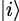)i=1,...,N and (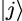)j=1,...,M denote, respectively, the standard orthonormal bases of the Hilbert spaces of systems A and B: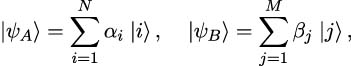where (αi)i=1,...,N and (βj)j=1,...,M are some probability amplitudes. The states that allow the state vector representation (1.3.1) are called pure states. In this case, the state of the combined system is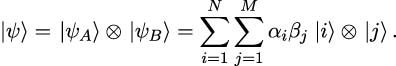However, in general, the state of the combined system would look like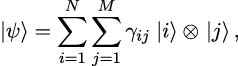where γij are probability amplitudes that may not necessarily be factorised as the product of probability amplitudes (αi)i=1,...,N and (βj)j=1,...,M. If γij cannot be factorised as αiβj, then the component systems A and B are entangled and their states cannot be represented by the state vectors (1.3.1). Such states of systems A and B are called mixed states.

The more general setup is that of an ensemble of states of the form {pk,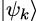}k=1,…,N, where each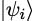is a quantum state whose wavefunction is known with certainty (although this does not necessarily provide full knowledge of the measurement statistics), and each pk is the associated probability (not amplitude) in [0,1]. In order to define properly pure and mixed states, introduce the density operator as follows:

Definition 7. A density operator ρ is a positive semidefinite Hermitian operator with unit trace and takes the form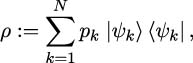where k=1Npk = 1 and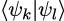equals 1 if k = l and zero otherwise.

Mathematically, such a density operator ρ corresponds to a density matrix (ρkl)k,l=1,…,N such that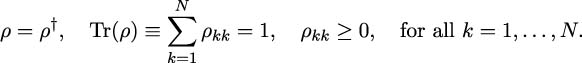## 1.3.2 Pure state

A pure state is one that can be represented by a state vector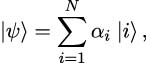where (αi)i=1,...,N are probability amplitudes in such that i=1N|αi|2 = 1. In the ensemble setup above, this means that there exists k∈{1,…,N} such that pk = 1 and hence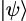=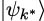and therefore ρ =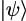ψ|. The density matrix also allows us to compute expectations of the form (1.2.4):

Lemma 3. Let ρ be the density matrix associated to the pure state (1.3.2) and let 𝒜 be an observable (Hermitian operator), then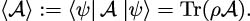Proof. The lemma follows from the immediate computation

 ⟨ψ|𝒜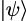= ⟨ψ|𝒜∑ i=1Nα i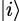= ∑ i=1Nα i ⟨ψ|𝒜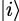= ∑ i=1N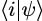⟨ψ|𝒜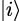= ∑ i=1N ⟨i|ρ𝒜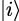= Tr(ρ𝒜).

With the state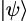given by (1.3.2), we obtain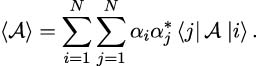At the same time we have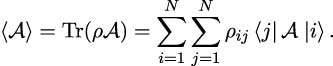Comparison of (1.3.2) and (1.3.2) yields the following expression for the density matrix of a pure state: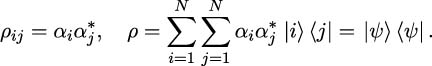Example: An example of a pure state is the Hadamard state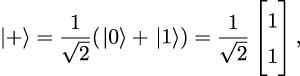with corresponding density matrix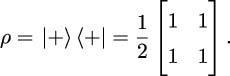## 1.3.3 Mixed state

A mixed state is one that cannot be represented by a single pure state vector, and is therefore represented as a statistical distribution of pure states in the form of an ensemble of quantum states {pk,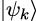}k=1,…,N, where k=1Npk = 1 and pk [0,1] for each k. The density of a mixed state therefore reads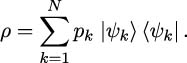Similarly to Lemma 3, we can write expectations of observables with respect to mixed states using the density matrix:

Lemma 4. Let ρ be the density matrix associated to the mixed state (1.3.3) and let 𝒜 be an observable (Hermitian operator), then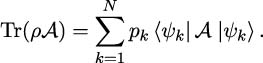Proof. The lemma follows from the immediate computation

 Tr(ρ𝒜) = ∑ i=1N ⟨i|ρ𝒜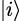= ∑ i=1N ⟨i|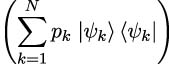𝒜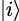= ∑ k=1Np k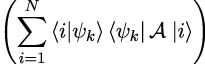= ∑ k=1Np k ⟨ψk|𝒜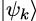.

Let us see now how the density matrix formalism can help us describe the state of a combined system. Consider an entangled state of two systems, A and B, given by (1.3.1), and a Hermitian operator 𝒜 that only acts within the Hilbert space of system A. What would be the expectation value of 𝒜 in this state? Starting with (1.2.4), we obtain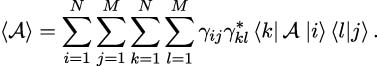Since only terms with l = j survive in (1.3.3) due to the orthogonality of the basis states, we have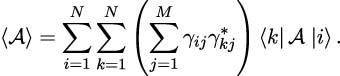Thus, the density matrix that describes the mixed state of system A is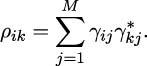Note that in the case where the probability amplitudes γij can be factorised as the product of probability amplitudes (αi)i=1,...,N and (βj)j=1,...,M, we obtain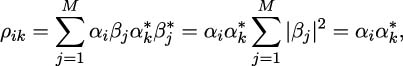which describes a pure state.

A simple criterion to distinguish a pure state from a mixed state is the following:

Lemma 5. Let ρ be a density matrix. The inequality Tr(ρ2) 1 always holds and Tr(ρ2) = 1 if and only if ρ corresponds to a pure state.

Proof. Consider an ensemble of pure states {pi,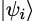}i=1,…,N, with density matrix given by (1.3.3). Therefore

 Tr(ρ2) = Tr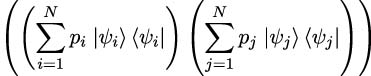= Tr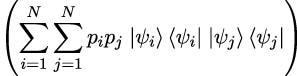= Tr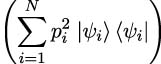= ∑ i=1Np i2Tr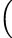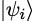⟨ψi|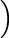= ∑ i=1Np i2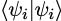= ∑ i=1Np i2,

which is smaller than 1 since the pi are probabilities in [0,1] summing up to 1. Assume now that Tr(ρ2) equals one, then so does i=1Npi2. If pi (0,1) for all i = 1,…,N, then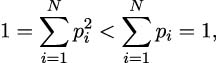which is a contradiction, and therefore there exists i∈{1,…,N} such that pi = 1, so that ρ =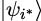ψi| is a pure state. Conversely, if ρ =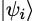ψi| for some i ∈{1,…,N} represents a pure state, then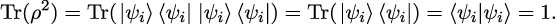Example: An example of a mixed state is a statistical ensemble of states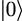and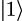. If a physical system is prepared to be either in state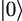or state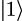with equal probability, it can be described by the mixed state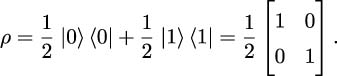Note that this is different from the density matrix of the pure state